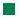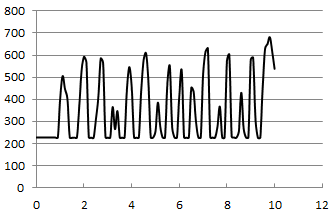TigerJython4Kids robotics Robotik
datenerfassung
 Deutsch   English

## 10. DATA ACQUISITION### YOU LEARN HERE...

 how to use the micro:bit as a data acquisition device that records and stores readings and how to transfer stored data to a PC..

###To capture a physical quantity and process it digitally, you need a sensor that converts the physical value into a voltage. Normally, this is an analog voltage that can take any value within a certain range. To use it in a computer system it has to be converted into a number (integer) by an analog-to-digital converter (ADC). The micro:bit pin P0 can be configured as input of an ADC that converts voltages 0..3.3V to numbers in the 10-bit range 0..1023. The command pin0.read_analog() performs the conversion and returns the number.

### EXAMPLES

 For your first experience, you want to investigate how well your fingers conduct electricity. You touch with the index finger the 3V pin and with the middle finger pin P0. In your program you perform a measurement every 100 ms and write the current time (in 1/10s) and the measured value into the console.Program: ```from microbit import * t = 0 while True: v = pin0.read_analog() print("t = %4.1f v = %d" %(t, v)) t += 0.1 sleep(100) ``` from microbit import * t = 0 while True: v = pin0.read_analog() print("t = %4.1f v = %d" %(t, v)) t += 0.1 sleep(100) ► Copy to clipboard In the print() function you use an elegant formatting specification, so that the values are automatically rounded to the desired number of digits. For example the format string %4.1f says that you want to output a field of length 4 with a decimal number (float) that is rounded to 1 decimal place. %d is the format string for an integer. The values themselves then follow the string in a parenthesis with a preceding % sign. In a typical measurement equipment you want to record a series of measurements and transfer them later to a computer for further data processing and visualization. Such a system is also called a data logger, because you log the acquired data in a log file. To do this you simply have to open a text file with open('data.log') and store the data strings s with f.write(s). For convenience, the fields t and v are separated by a semicolon. Don't forget to add a new line character \n to separate the records line-by-line. You start the data recording for 10 s by clicking the button A. Program: ```from microbit import * display.show(Image.SQUARE_SMALL) while not button_a.was_pressed(): sleep(10) display.show(Image.SQUARE) T = 10 with open('data.log', 'w') as f: t = 0 while t < T: v = pin0.read_analog() f.write("%4.1f; %d\n" %(t, v)) t += 0.1 sleep(100) display.show(Image.NO) ``` from microbit import * display.show(Image.SQUARE_SMALL) while not button_a.was_pressed(): sleep(10) display.show(Image.SQUARE) T = 10 with open('data.log', 'w') as f: t = 0 while t < T: v = pin0.read_analog() f.write("%4.1f; %d\n" %(t, v)) t += 0.1 sleep(100) display.show(Image.NO) ► Copy to clipboard To transfer the data to a PC you can use the module mbm of TigerJython and extract the file with the command extract() from the micro:bit and copy it to the directory where the program is located. Since this is a Python program that runs on the PC, you have to click the green Run button and not the black Download/Execute button. ```from mbm import * extract('data.log') ``` Use any text editor and open the log file to check the data. You may visualize them with a spreadsheet program or another graphics tool (such as TigerJython's GPanel).### MEMO

 A data logger converts the measurement data into a digital number and stores it in a file, usually by adding a time stamp.

### EXERCISES

1.
 Write the accelerometer readings to a file and plot the result graphically. For example, record the magnitude of acceleration every 20 milliseconds for 2 seconds while you shake the micro:bit. You can even investigate the free fall of an object.
2.
 You want to capture the brightness change of ambient light. Get a photoresistor (Light Dependent Resistor, LDR) from an electronic store and connect it with a resistance of 1 kOhm in the following circuit. For example, record the brightness every 0.1 s for 10 s and graph it.3 You can check in the console window if the log file has been successfully created. To do this, type after the Python prompt: >>> from os import * Now the functions of the module os are available to you. You can list them with >>> dir(os) and print the names of all files in the local file system with >>>listdir() To show the size of the log file, type >>> size('data.log')

3-1

Acceleration generally means the rate of change of velocity, that is, one-dimensionalwhere v is the speed.

3-2

From the three acceleration components you can calculate pitch and roll angles as shown below. Start the following program and tilt the board sideways or forwards and backwards. In the terminal window, the values of pitch and roll are written out in degrees.

```from microbit import *
from math import *

def getPitch():
a = accelerometer.get_values()
pitch = atan2(a, -a)
return int(degrees(pitch))

def getRoll():
a = accelerometer.get_values()
anorm = sqrt(a * a + a * a + a * a)
roll = asin(a / anorm)
return int(degrees(roll))

while True:
pitch = getPitch()
roll = getRoll()
print("p: " + str(pitch))
print("r: " + str(roll))
print()
sleep(100)
```